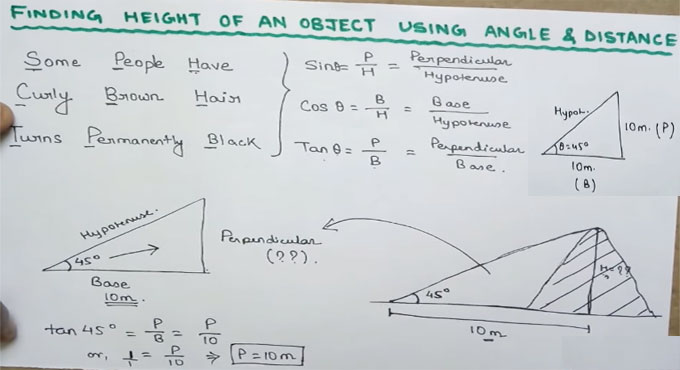# How to calculate the height of an object on the basis of given angle & distanceBy watching this construction video tutorial, you will be able to work out the height of any object on the basis of angle and distance provided. It is very useful for surveyors.

In brief, the height of an object is determined by calculating the distance from the object and the angle of elevation of the top of the object.

The tangent of the angle refers to the object height that is divided by the distance from the object. In this way, the height is obtained.

The calculation is made on the basis of the following formula :-

Sin? = P/H = Perpendicular/Hypotenuse

Cos? = B/H = Base/Hypotenuse

Tan? = P/B = Perpendicular/Base

Suppose, in a triangle, the angle is taken as 45 degree. The opposite of the angle should be always taken as perpendicular and opposite site of the perpendicular should be taken as hypotenuse. The horizontal line is taken as base and it?s measurement is 10 meter.

Now, all the values should be put on the above formulas to check which one is matched to determine the height. Watch the following video tutorial to learn the remaining calculation process.